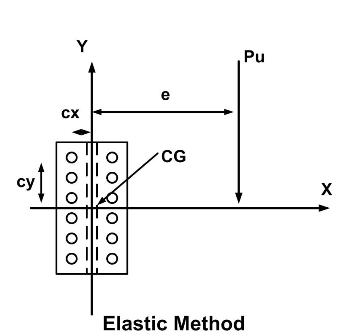* Log In to use the Calculate function * Become a Member!

Calculate Shear Force on a Bolt With Eccentric Loading in Faying Surface Plane:

Calculation:
Designer/Checker:

Input:(Note: All input Metric units are converted to English units for the equations below and then the output English units are converted back to Metric)

AISC Chapter 7:
The following uses the elastic method for calculating shear force of the most stressed bolt in a connection with eccentric loading in the plane of the faying surface.

Distance from the center of gravity of bolt group to the direct shear force (e - in or mm)
Direct shear force (Pu - kips or kN)
Number of bolts in the connection (n)
Polar moment of inertia of the bolt group (Ip - in^4 or mm^4)
Distance from the y-axis of the center of gravity, CG,of the bolt group to the bolt (cx - in or mm)
Distance from the x-axis of the center of gravity, CG, of the bolt group to the bolt (cy - in or mm)
Angle of the applied shear from y-axis of the bolt group, (Ang - degrees)

Output: